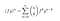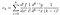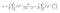# Abstract

Following from the previous article I had written on the Taylor series here, in this article, I present a method for deriving the Leibniz product rule from Taylor’s theorem and Cauchy product rule.

# Introduction

The Leibniz rule gives us a nice closed form for the nth derivative of the product of two functions as a summation of binomial coefficients and derivatives of the individual functions:The exponent denotes derivatives, so the ’n’ on the left side means the nth derivative of the product

Almost looks like a binomial theorem for expanding derivatives, doesn’t it? I wonder if there is a math field that studies sp00ky relationships such as these.

# Cauchy Product Rule

The Cauchy product rule, in the context of this article, gives a nice way to find the product of two infinite polynomial series. For illustrating, let’s consider two infinite polynomial series represented as P and Q defined the following way:

Now the multiplication of these two series would produce another infinite polynomial but this time with some other coefficients:

Now the only challenge is relating the coefficients of the polynomial series in the product i.e : a_i, b_j, and the coefficient of the polynomial series resulted from multiplication c_k .

This may look intimidating, but it’s not too hard. We simply have to sum the product a_i *b_j over all the i,j pairs which satisfy the equation: i+j=k since those are all the indice pairs produce x^k in the product

But, with a little bit of cleverness, we could rewrite the sum in terms of a single index by writing j=k-i,

Great, now we have ‘multiplied’ the two polynomial series and… it’s time to combine set up Taylor’s theorem in a way we can apply this to get the Leibniz rule. Onwards!

# Combining Taylor series and Cauchy

Let’s consider two ‘nice’ functions which are well behaved (unlike this naughty one) with their Taylor expansions around some point x=a,

And the product of the two above could be written as another polynomial series (x-a) as so:

Now, this is where we unleash the ‘Cauchy’ because if you look at carefully, the same arguments we used for reaching the Cauchy formula apply here with the only difference being here it’s an (x-a) instead of ‘x’. From (4), the coefficients are:(8) Everything is evaluated at x=a, but it’d be too cluttered if I put that in as well, so I dropped the evaluation.

We will switch over to the nicer and aesthetically pleasing notation of denoting the number of times we took the derivative on a function by the exponent on the function. Rewriting the (8) with the new notation:

Now that’s elegant but it just looks like the formula is begging us to keep a k! in the place of that one so that we can get the binomial coefficient out, so let’s just do that:(10) The k! is independent of the summation, so we can take it out

Now that we are done beautifying the math, let’s go back to working our original proof. In (7), we could directly write a Taylor polynomial for the product of the two functions:

Now, if the polynomial series (11) and (7) are equal then then coefficients of each monomial term in the individual polynomial series should be the same! Hence, we can write :

But but but, we had just derived a ‘nice’ expression for c_k in (9), using that:

Cancelling of factorial in the denominator of both sides, ditching the evaluation (x=a thing), we arrive at the general Leibniz theorem for differentiating product of two functions:

Pretty cool, eh?

# Applications

Suppose we wanted to do a ‘hundredth derivative’, perhaps something of the sort below:

We can just plug and chug this into the formula:

Considering that the derivative of the exponential function is it self, and also noting that all higher derivatives beyond the third derivative of x³ are zeros, we find that the summation’s upper index is till three as all after are zeros.

Now, if we use the ‘nth’ derivative of monomial formula in the original Taylor series article, we will arrive at :

Expanding this with a simple summation gives our answer. Taking a step back and seeing the big picture, we see that the Leibniz rule tells us how to easily evaluate the nth derivative of a product of two functions from the formulas for the nth derivative of each function in the product.

And all there is to it! Hope you all enjoyed reading this article :) Bonus: Try deriving an extended leibniz product rule for product of three or more functions.. perhaps a multinomial theorem analogy would apply?